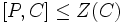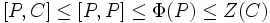# Abelian Frattini subgroup implies centralizer is critical

## Statement

Suppose$P$ is a group of prime power order such that the Frattini subgroup (?)$\Phi(P)$ of$P$ is an Abelian group (?). Then, the centralizer of the Frattini subgroup, i.e., the group$C_P(\Phi(P))$, is a Critical subgroup (?) of$P$.

Note that the critical subgroup we obtain this way is of a special kind: it is a C-closed critical subgroup (?).

## Definitions used

### Frattini subgroup

Further information: Frattini subgroup

The Frattini subgroup of a group is defined as the intersection of all its maximal subgroups. For a$p$-group, it is the unique smallest group such that the quotient is elementary Abelian.

In particular, for a$p$-group, the Frattini subgroup contains the commutator subgroup, and it also contains the Frattini subgroup of any intermediate subgroup.

### Critical subgroup

Further information: Critical subgroup

A subgroup$C$ of a group$P$ is termed critical in$P$ if$C$ is a characteristic subgroup of$P$ and the following hold:

1.$\Phi(C) \le Z(C)$; In other words,$C$ is a Frattini-in-center group.
2.$[P,C] \le Z(C)$: In other words,$C$ is a commutator-in-center subgroup of$P$.
3.$C_P(C) \le C$: In other words,$C$ is a self-centralizing subgroup of$P$.

## Facts used

1. Frattini subgroup is characteristic
2. Characteristicity is centralizer-closed: The centralizer of a characteristic subgroup is characteristic.

## Proof

Given: A finite$p$-group$P$ with Abelian Frattini subgroup$\Phi(P)$.$C = C_P(\Phi(P))$.

To prove:$C$ is a critical subgroup of$P$.

Proof: By facts (1) and (2),$C$ is characteristic in$P$. Note also that since$\Phi(P)$ is Abelian,$\Phi(P) \le C$.

We now check each condition:

1.$\Phi(C) \le Z(C)$: First, observe that$\Phi(C) \le \Phi(P)$, because$C/\Phi(P)$ is elementary Abelian. By definition of$C$, we have$\Phi(P) \le Z(C)$. Thus,$\Phi(C) \le Z(C)$.
2.$[P,C] \le Z(C)$: Indeed,$[P,C] \le [P,P] \le \Phi(P) \le Z(C)$.
3.$C_P(C) \le C$: We have$C_P(C) \le C_P(\Phi(P))$, because$\Phi(P) \le C$. Thus,$C_P(C) \le C$.